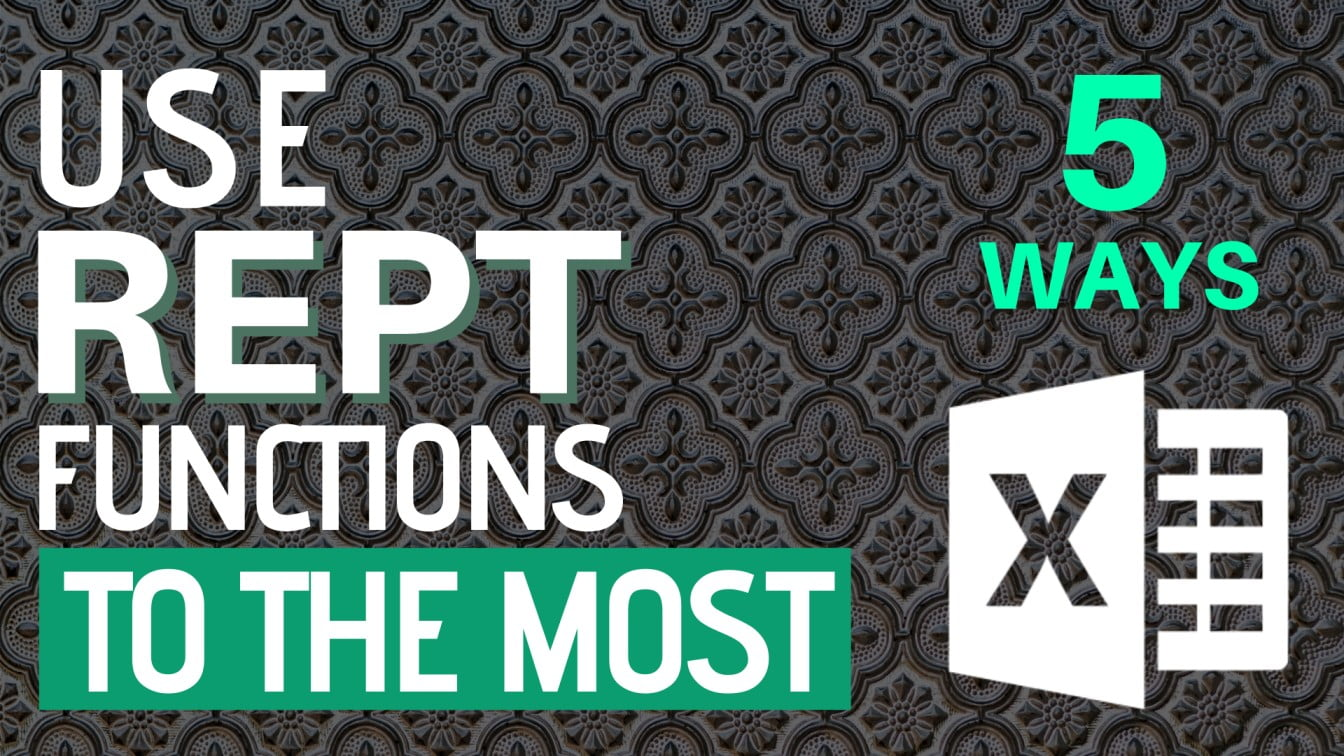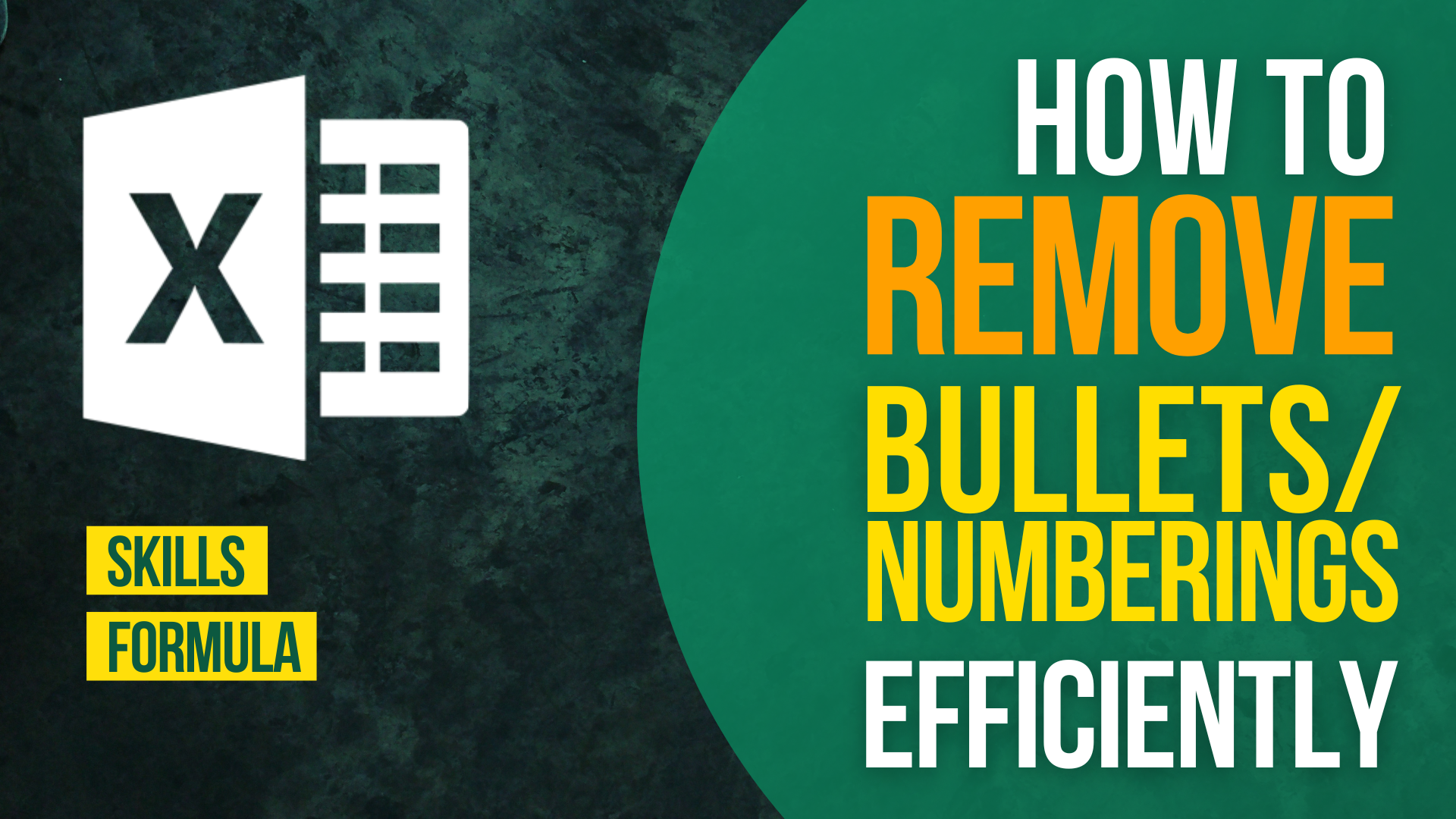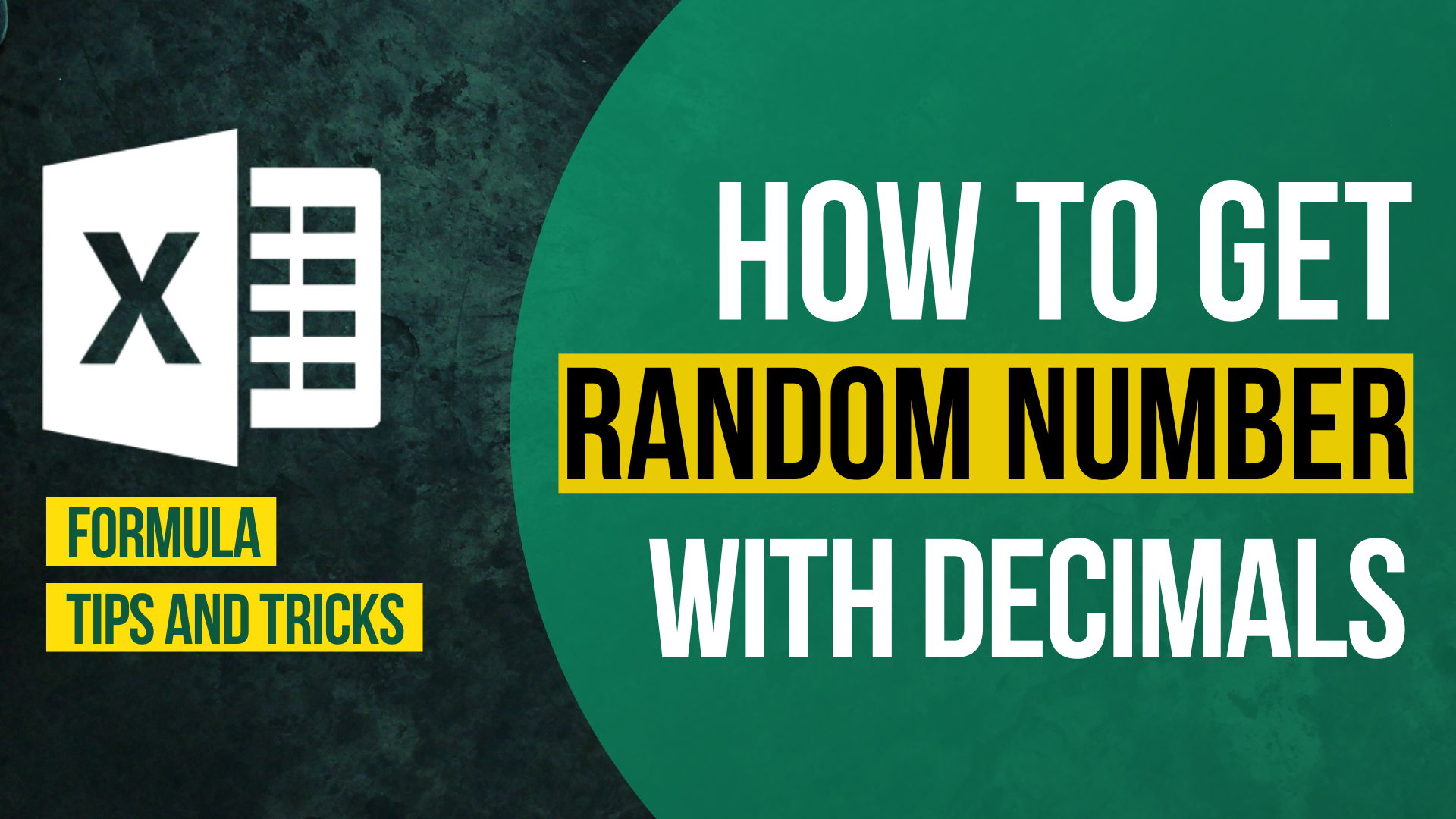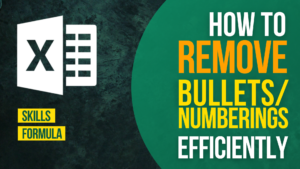# How to use REPT functions to the Most## Repeat a set of character

Formula of Cell C2

`=REPT(A2,B2)`

=REPT(character to repeat, the number of times you would like it to repeat)

This is the most common usage of REPT. Specify the character to repeat and the number of times you would like it to repeat and there you go the results.

## Equalise length of number string

Formula of Cell B2

`=REPT(0,5-LEN(A2))&A2`

Sometimes we may want to equalise length of the number string. Apart from tweaking the number format, REPT function can also do us a favor.

Say you want a 6-digit number. You can change the “5” in the above formula. The new formula should look like this.

`=REPT(0,6-LEN(A2))&A2`

You might also be interested in How To Do Case-sensitive Find And Replace In Excel?

## Create in-cell charts

You can use REPT to create different types of in-cell charts, such as mirror graph, star-rating scheme and more.

Aren’t they stunning?

Learn how to create those charts by reading 5 Types of Graphs to Create with REPT function.

## Extract words from a text string

Formula of Cell B2

`=TRIM(RIGHT(SUBSTITUTE(A2," ",REPT(" ",LEN(A2))),3*LEN(A2)))`

You might also be interested in How To Reverse Concatenate In Excel (3 ways)

## Set Row height

Formula of Cell B2

`=REPT(CHAR(10),1)`

Resizing row height is not as easy as resizing column width. However, if you fail to adjust the row height, some text in cells may be collapsed and cannot be seen.

Using the above formula allows you to determine how tall you would like the row to be.

The CHAR returns a character when receiving a character code. Char(10) returns a line break.

So =REPT(CHAR(10),1) will return one line break, =REPT(CHAR(10),2) will return two.

Hungry for more useful Excel tips like this? Subscribe to our newsletter to make sure you won’t miss out on any of our posts and get exclusive Excel tips!How to Input Time Duration in ExcelHow to Remove Bullets/Numberings in Excel

Popular posts